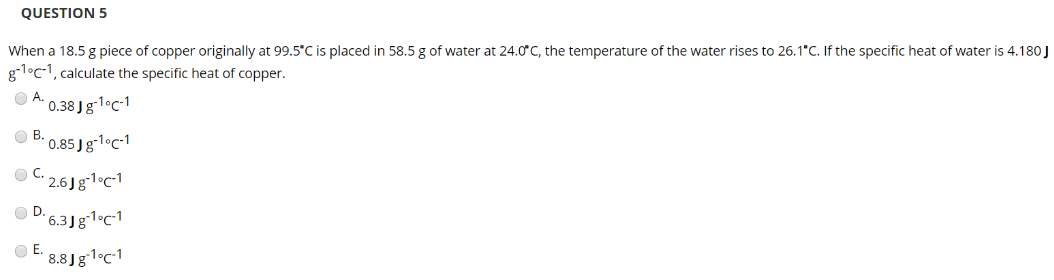# When a 18.5 g piece of copper originally at 99.5°C is placed in 58.5 g of water at 24°C rises to 26.1°C. If the specific heat of water is 4.180 J g^-1°C^-1, calculate the specific heat of copper. A. 0.38 J g^-1°C^-1 B. 0.85 J g^-1°C^-1 C. 2.6 J g^-1°C^-1 D. 6.3 J g^-1°C^-1 E. 8.8 J g^-1°C^-1